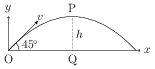# Angular Momentum

## Problems from IIT JEE

Problem (IIT JEE 1990): A particle of mass $m$ is projected with a velocity $v$ making an angle of $45^\mathrm{o}$ with the horizontal. The magnitude of the angular momentum of the projectile about the point of projection when the particle is at its maximum height $h$ is,

1. zero
2. $\frac{mv^3}{4\sqrt{2} g}$
3. $\frac{mv^3}{\sqrt{2} g}$
4. $m\sqrt{2gh^3}$

Solution:At the highest point P, vertical component of the velocity becomes zero. The maximum height is given by, \begin{align} h={v^2\sin^2 45}/{(2g)}={v^2}/{(4g)}.\nonumber \end{align} The position vector of P and velocity at P are, \begin{align} &\vec{r}_P=|\mathrm{OQ}|\,\hat\imath+h\,\hat\jmath,\nonumber\\ &\vec{v}_P=v \cos45\,\hat\imath={v}/{\sqrt{2}}\;\hat\imath.\nonumber \end{align} The angular momentum about O is given by, \begin{align} &\vec{L}=m\vec{r}_P\times\vec{v}_P=-{mvh}/{\sqrt{2}}\,\;\hat{k},\nonumber\\ &|\vec{L}|={mvh}/{\sqrt{2}}={mv^3}/{4\sqrt{2}g}=m\sqrt{2gh^3}.\nonumber \end{align}